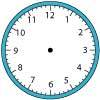#### You may also like### Right Time

At the time of writing the hour and minute hands of my clock are at right angles. How long will it be before they are at right angles again?### Isosceles

Prove that a triangle with sides of length 5, 5 and 6 has the same area as a triangle with sides of length 5, 5 and 8. Find other pairs of non-congruent isosceles triangles which have equal areas.Four rods, two of length a and two of length b, are linked to form a kite. The linkage is moveable so that the angles change. What is the maximum area of the kite?

# Inner Rectangle

##### Age 11 to 14 Short Challenge Level:

If PQRS is a rectangle, then the angle at P is a right angle, and so the opposite angle at P is also a right angle, as shown in the diagram below. Since the lines drawn are angle bisectors, the green angles are equal, the orange angles are equal and the blue angles are equal.By triangle ABP, the green angle and the orange angle must add up to 90$^\text{o}$.

Similarly, from triangle AQD, which has a right angle at Q, the blue angle and the orange angle must add up to 90$^\text{o}$.

So the green and the blue angles must be the same. Continuing, the angles at A and C must also be equal, so opposite angles of ABCD are equal. Which means that ABCD must be a parallelogram.
You can find more short problems, arranged by curriculum topic, in our short problems collection.